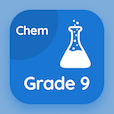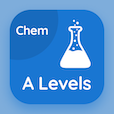SAT Prep Courses

SAT Chemistry MCQs

SAT Chemistry MCQ - Topics

Practice Empirical Formula Multiple Choice Questions (MCQ Quiz), Empirical Formula quiz answers PDF to study sat chemistry course for sat chemistry online classes. Mole Concept Multiple Choice Questions and Answers (MCQs), Empirical Formula quiz questions for graduate school interview questions. Empirical Formula Book PDF: empirical formula, molar mass test prep for free career quiz.

"In combustion analysis, % of hydrogen is calculated from" MCQ PDF: empirical formula App APK with ch, co, h2o, and co2 choices for graduate school interview questions. Learn empirical formula quiz questions for merit scholarship test and certificate programs for online high school college acceptance.

## MCQ on Empirical Formula Quiz

MCQ: In combustion analysis, % of hydrogen is calculated from

CH
CO
H2O
CO2

MCQ: In combustion analysis, % of hydrogen is calculated from

C6 H6
CH
CHO
CO2

MCQ: The formula mass of an ionic compound is called

Gram formula
Simple formula
Empirical formula
Molecular formula

MCQ: Empirical formula of glucose is

CHO
CH2O
C2HO
CHO2

MCQ: In case of ionic compounds, the molecular and empirical formulas are

Same
Different
Not same
All of above

### More Quizzes on SAT Chemistry Book